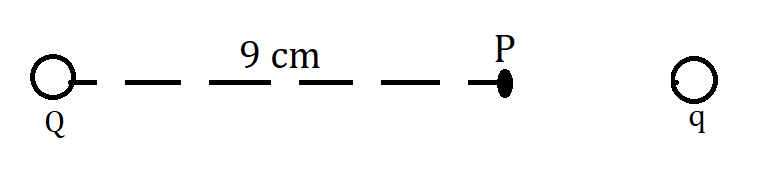Home/Class 12/Physics/

Calculate the potential at a point  $$P$$  due to a charge of  $$4\times 10^{-7}C$$  located  $$9\text{ cm}$$  away. Hence obtain the work done in bringing a charge of  $$2\times 10^{-9}C$$  from infinity to the point  $$P$$ . Does the answer depend on the path along which the charge is brought?Speed
00:00
01:52## QuestionPhysicsClass 12

Calculate the potential at a point  $$P$$  due to a charge of  $$4\times 10^{-7}C$$  located  $$9\text{ cm}$$  away. Hence obtain the work done in bringing a charge of  $$2\times 10^{-9}C$$  from infinity to the point  $$P$$ . Does the answer depend on the path along which the charge is brought?

See analysis below
4.64.6## Solution

Given :$$Q=4\times 10^{-7}C,r=0.09\;m$$
For source charge, Potential at  $$P$$  due to  $$Q$$  charge,
$$V_P=\left(\frac 1{4\pi \varepsilon _0}\right)\left(\frac Q r\right)$$
$$=\frac{\left(9\times 10^9\right)\left(4\times 10^{-7}\right)}{0.09}$$
$$=4\times 10^4\;V$$
Given data,
Test charge, $$q=2\times 10^{-9}C$$
Potential at point  $$P=$$   $$4\times 10^4\;V$$
Potential at a point (test charge) is the amount of work done to bring unit charge from infinity to that point.
$$W_{{\infty}P}=qV_p$$
$$=2\times 10^{-9}\times 4\times 10^4$$
$$=8\times 10^{-5}\;J$$
No, this work done does not depend on the path followed. The reason is that this work is against electrostatic force which is a conservative force.